# 动态规划的实际应用：图片压缩算法 6月15日 【今日算法】 -问答-阿里云开发者社区-阿里云

## 动态规划的实际应用：图片压缩算法 6月15日 【今日算法】游客ih62co2qqq5ww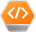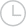2020-06-17 02:16:53409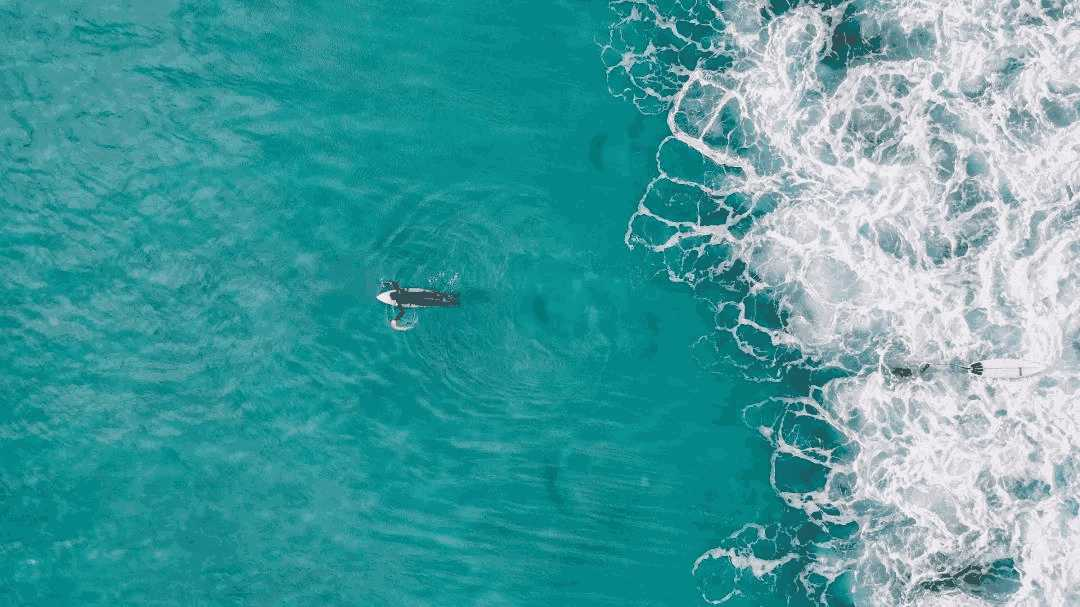Avidan 和 Shamir 在他们的论文中展示的是一个叫做接缝裁剪的技术。它首先会识别出图片中不太有意义的“低能量”区域，然后找到穿过图片的能量最低的“接缝”。对于减少图片宽度的情况，接缝裁剪会找到一个竖向的、从图片顶部延伸到底部、下一行最多向左或向右移动一个像素的接缝。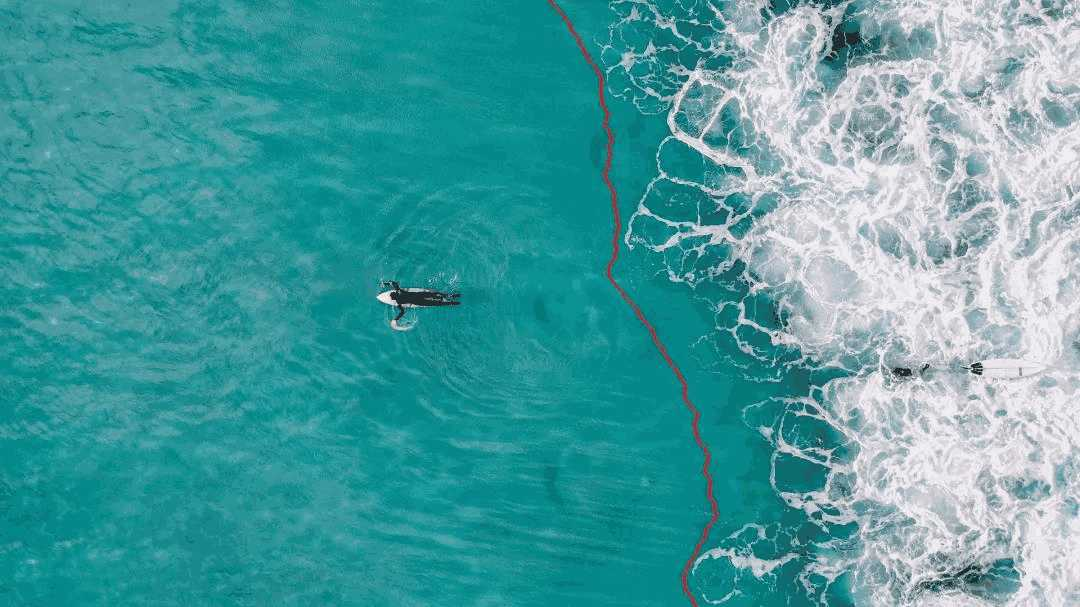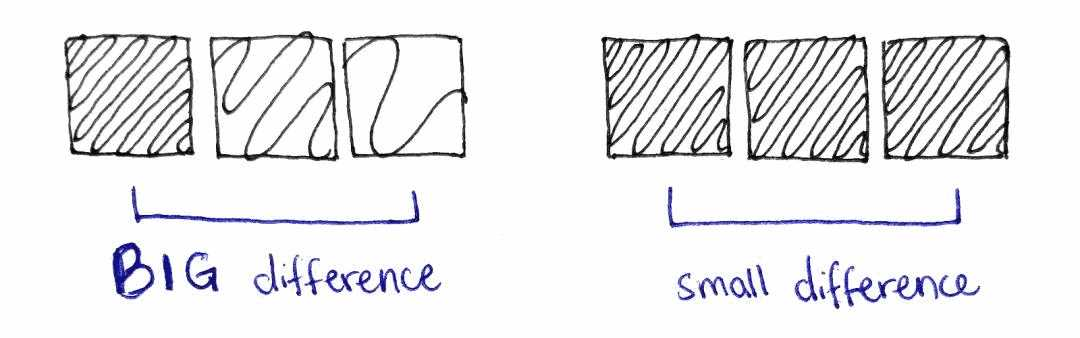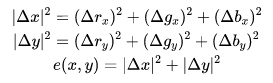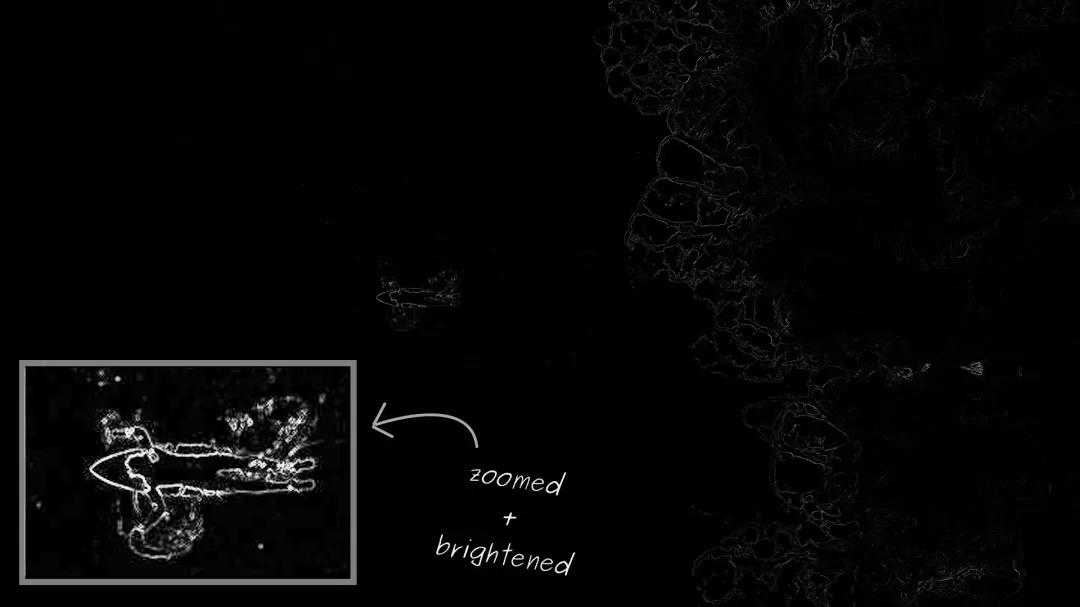• 接缝是像素的序列，其中每行有且仅有一个像素。要求对于连续的两行， 坐标的变化最多为 1，这保证了这是一条相连的接缝。
• 最低能量接缝是指接缝中所有像素的能量总和最小的一条接缝。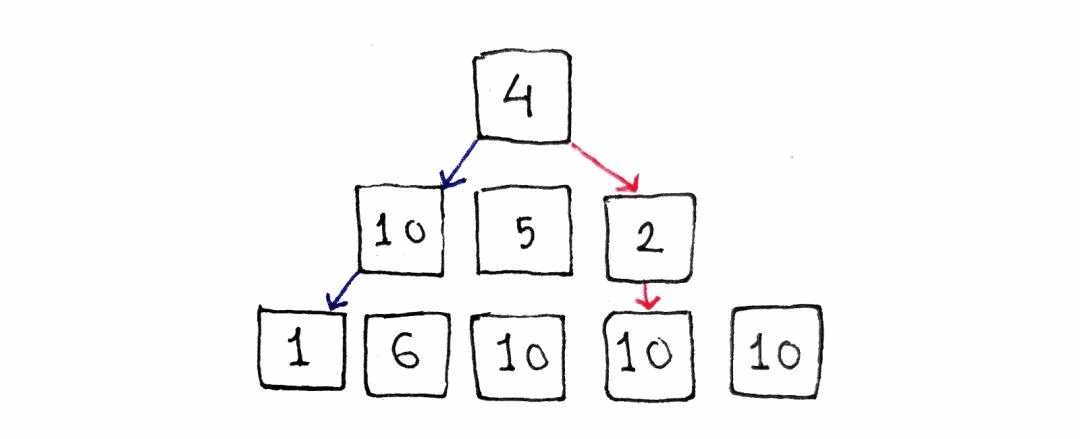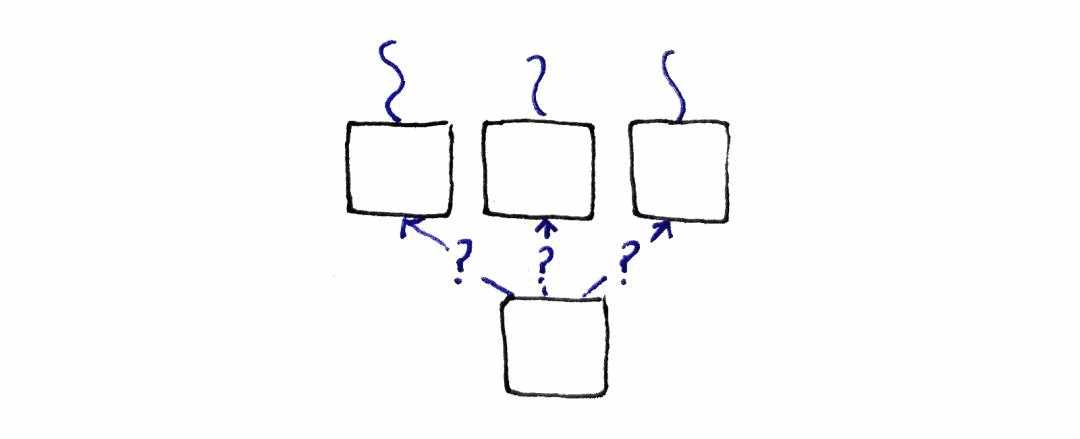M(x , 0)=e(x , 0)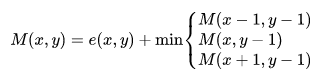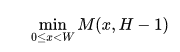• 递归关系的输入为整数。
• 我们所需的最终结果易于从递归关系中提取。
• 这个关系只依赖于自身。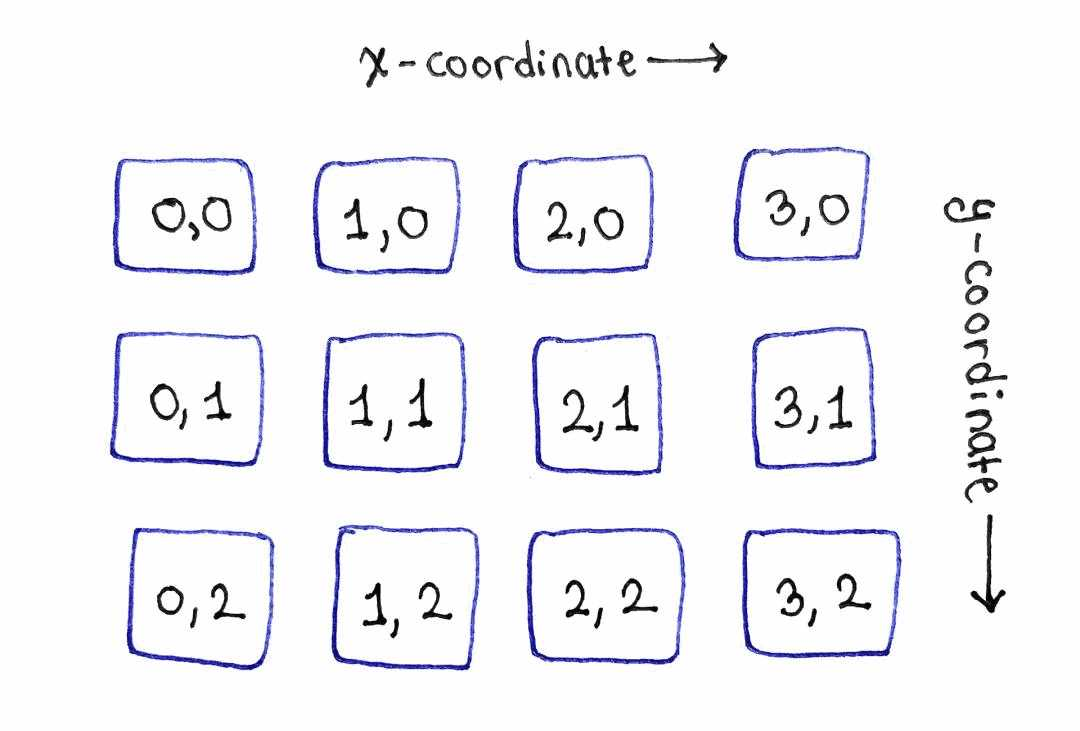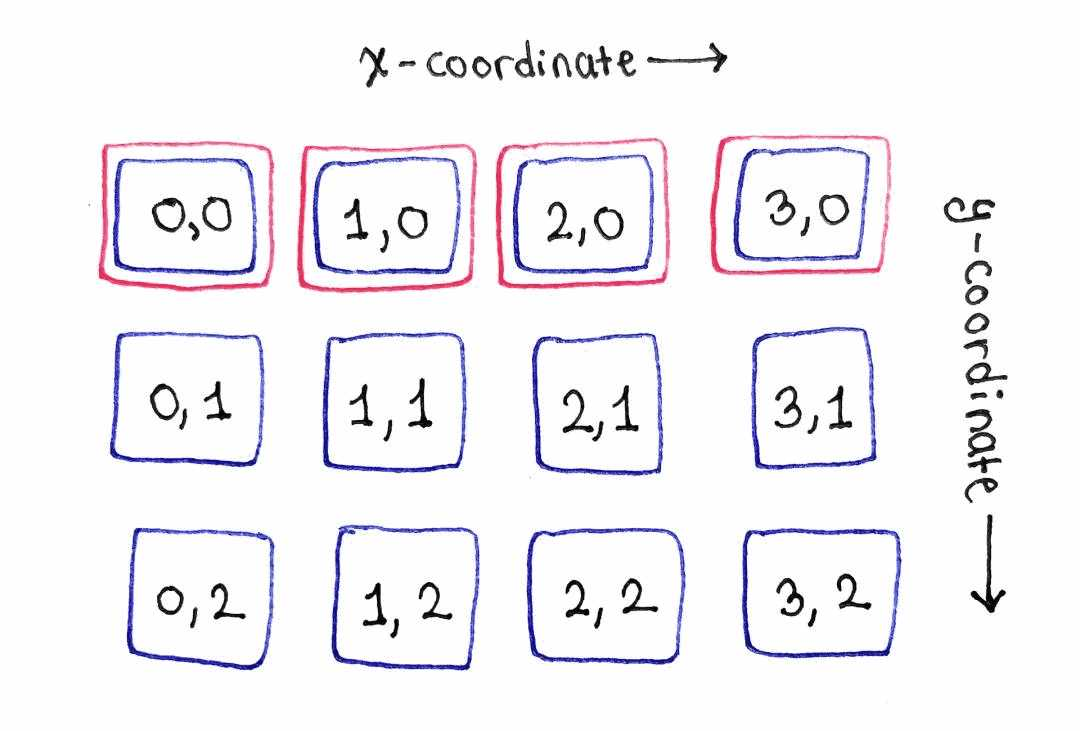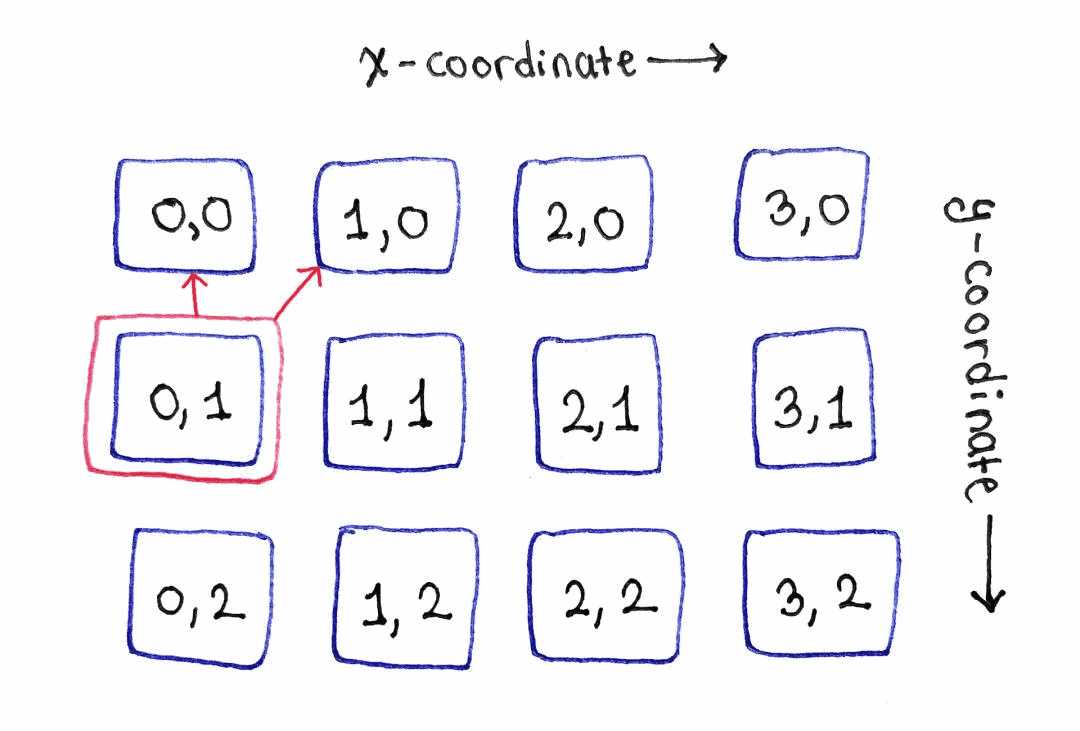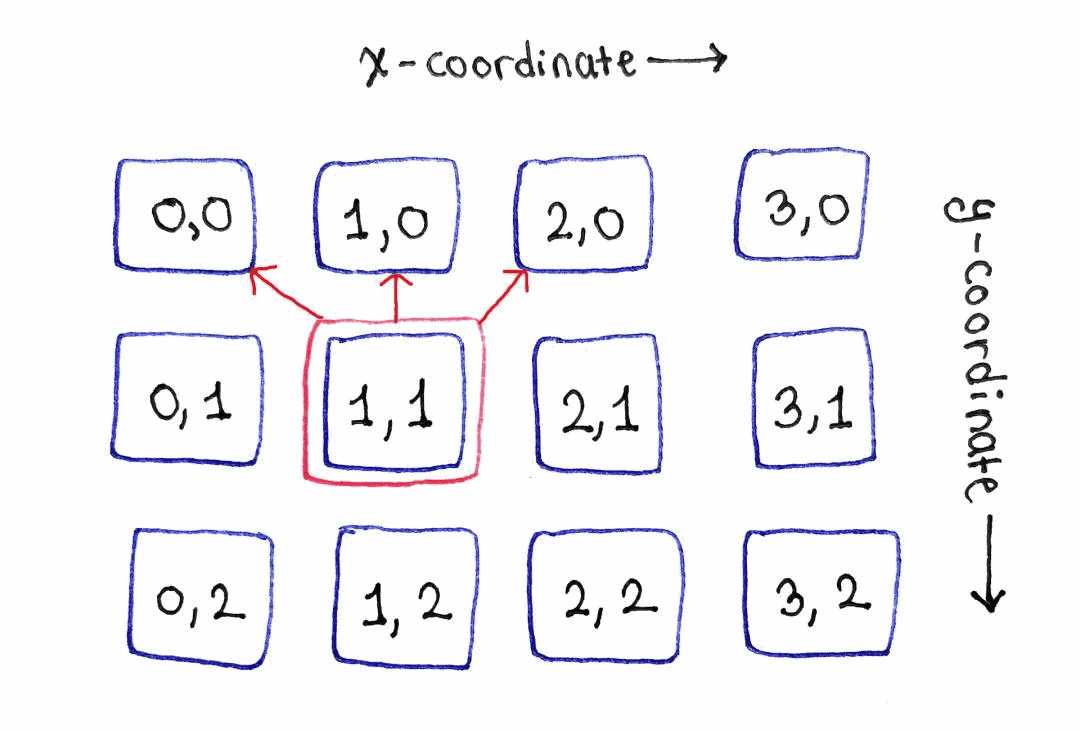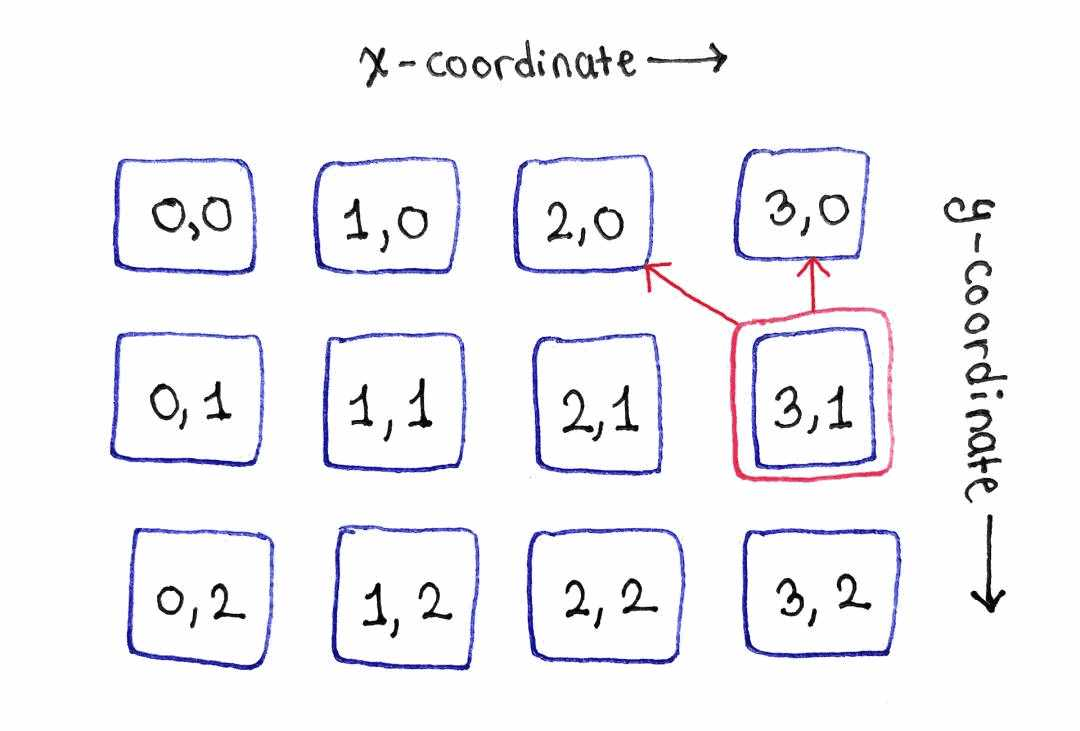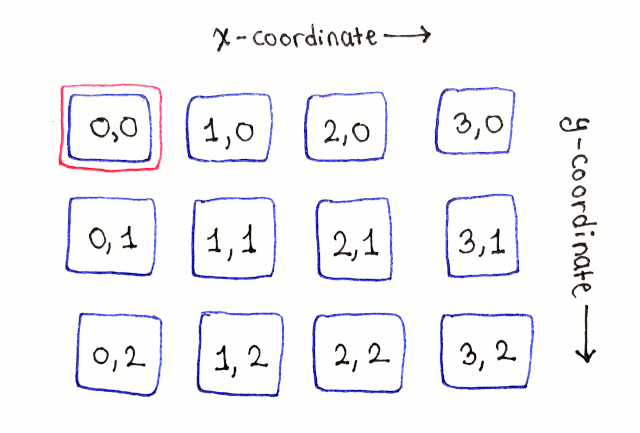• 从图片的顶部到底部。
• 对于每一行，可以以任意顺序。自然的顺序是从左至右。

``````previous_seam_energies_row = list(pixel_energies)
``````

``````# 在循环中跳过第一行
for y in range(1, len(pixel_energies)):
pixel_energies_row = pixel_energies[y]

seam_energies_row = []
for x, pixel_energy in enumerate(pixel_energies_row):
# 判断要在前一行中遍历的 x 值的范围。这个范围取决于当前像素是在图片
# 的中间还是边缘。
x_left = max(x - 1, 0)
x_right = min(x + 1, len(pixel_energies_row) - 1)
x_range = range(x_left, x_right + 1)

min_seam_energy = pixel_energy + \
min(previous_seam_energies_row[x_i] for x_i in x_range)
seam_energies_row.append(min_seam_energy)

previous_seam_energies_row = seam_energies_row
``````

``````min(seam_energy for seam_energy in previous_seam_energies_row)
``````

``````ENERGIES = [
[9, 9, 0, 9, 9],
[9, 1, 9, 8, 9],
[9, 9, 9, 9, 0],
[9, 9, 9, 0, 9],
]

print(min_seam_energy(ENERGIES))
``````

``````class SeamEnergyWithBackPointer():
def __init__(self, energy, x_coordinate_in_previous_row=None):
self.energy = energy
self.x_coordinate_in_previous_row = \
x_coordinate_in_previous_row
``````

``````seam_energies = []

# 拷贝最顶行的像素能量来初始化最顶行的接缝能量。最顶行没有后向指针。
seam_energies.append([
SeamEnergyWithBackPointer(pixel_energy)
for pixel_energy in pixel_energies
])
``````

• 前一行的数据包含的是 SeamEnergyWithBackPointer 的实例，所以当计算递归关系的值时，我们需要在这些对象内部查找接缝能量。
• 当为当前像素存储数据时，我们需要创建一个新的 SeamEnergyWithBackPointer 实例。在这个实例中我们既存储当前像素的接缝能量，又存储用于计算当前接缝能量的前一行的 坐标。
• 在每一行计算结束后，不会丢弃前一行的数据，而是简单地将当前行的数据追加到 seam_energies 中。
``````# 在循环中跳过第一行
for y in range(1, len(pixel_energies)):
pixel_energies_row = pixel_energies[y]

seam_energies_row = []
for x, pixel_energy in enumerate(pixel_energies_row):
# 判断要在前一行中遍历的 x 值的范围。这个范围取决于当前像素是在图片
# 的中间还是边缘。
x_left = max(x - 1, 0)
x_right = min(x + 1, len(pixel_energies_row) - 1)
x_range = range(x_left, x_right + 1)

min_parent_x = min(
x_range,
key=lambda x_i: seam_energies[y - 1][x_i].energy
)

min_seam_energy = SeamEnergyWithBackPointer(
pixel_energy + seam_energies[y - 1][min_parent_x].energy,
min_parent_x
)

seam_energies_row.append(min_seam_energy)

seam_energies.append(seam_energies_row)
``````

``````# 找到最底行接缝能量最低的 x 坐标
min_seam_end_x = min(
range(len(seam_energies[-1])),
key=lambda x: seam_energies[-1][x].energy
)
``````

``````# 沿着后向指针前进，得到一个构成最低能量接缝的坐标列表
seam = []
seam_point_x = min_seam_end_x
for y in range(len(seam_energies) - 1, -1, -1):
seam.append((seam_point_x, y))

seam_point_x = \
seam_energies[y][seam_point_x].x_coordinate_in_previous_row

seam.reverse()
``````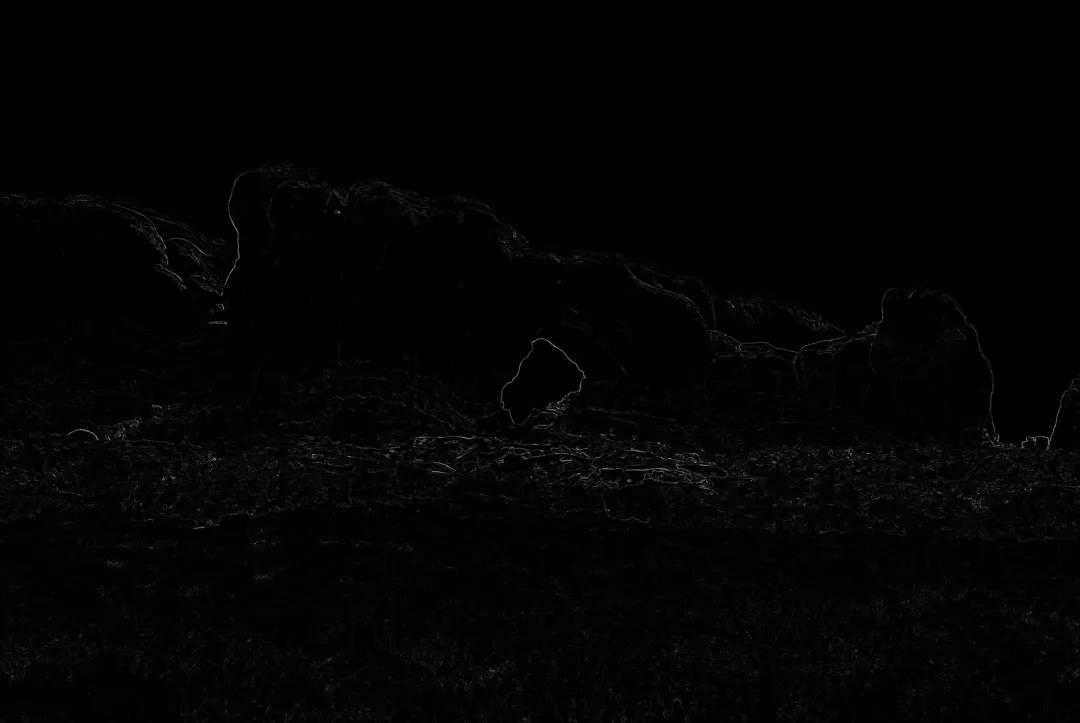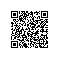取消 提交回答使用钉钉扫一扫加入圈子
+ 订阅